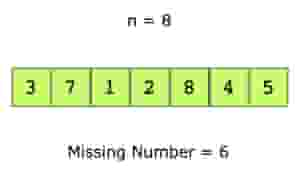# Find the Missing Number

## Problem Statement

You are given an array of positive numbers from 1 to n, such that all numbers from 1 to n are present except one number ‘x’. You have to find ‘x’. The input array is not sorted.

For example, let’s look at the below array.## Hint

• How would you calculate the sum of numbers from 1 to n?

## Try it yourself

int find_missing(const vector<int>& input) {
//TODO: Write - Your - Code
return -1;
}

## Solution

int find_missing(const vector<int>& input) {
// calculate sum of all elements
// in input vector
auto sum_of_elements = std::accumulate(
input.begin(), input.end(), 0);
// There is exactly 1 number missing
int n = input.size() + 1;
int actual_sum = (n * ( n + 1 ) ) / 2;
return actual_sum - sum_of_elements;
}

void test(int n) {
int missing_element = rand() % n + 1;
vector<int> v;
for (int i = 1; i <= n; ++i) {
if (i == missing_element) {
continue;
}
v.push_back(i);
}
int actual_missing = find_missing(v);
cout << "Expected Missing = " << missing_element << " Actual Missing = " << actual_missing << endl;
}

int main() {
srand (time(NULL));
for (int i = 1; i < 10; ++i)
test(100000);

return 0;
}

## Solution Explanation

Linear, O(n).

Constant, O(1).

### Solution Breakdown

A naive solution is to simply search for every integer between 1 and n in the input array, stopping the search as soon as there is a missing number. Since the input array is not sorted, its time complexity will be O($n^{2}$).

Can you do better than O($n^{2}$)? Yes.

You could sort the array and then do a linear scan O(n) where you compare all consecutive elements to find the missing number. However, the time complexity of this algorithm is O(n log n) due to the time spent in sorting.

You can do better. Here is a linear, O(n), solution that uses the arithmetic series sum formula.

Sum (1 - n) = $\frac{n (n + 1)}{2}$

​​Here are the steps to find the missing number.

1. Find the sum ‘sum_of_elements’ of all the numbers in the array. This would require a linear scan, O(n).
2. Then find the sum ‘expected_sum’ of first ‘n’ numbers using the arithmetic series sum formula i.e. ( n * (n + 1) ) / 2.
3. The difference between these i.e. ‘expected_sum - sum_of_elements’, is the missing number in the array.

Learn in-demand tech skills in half the time